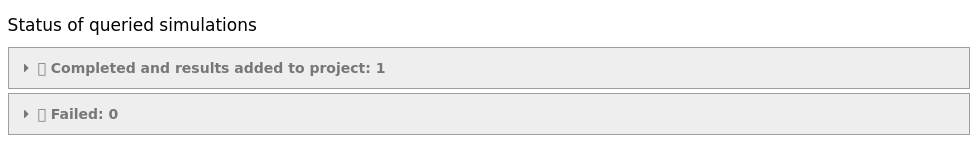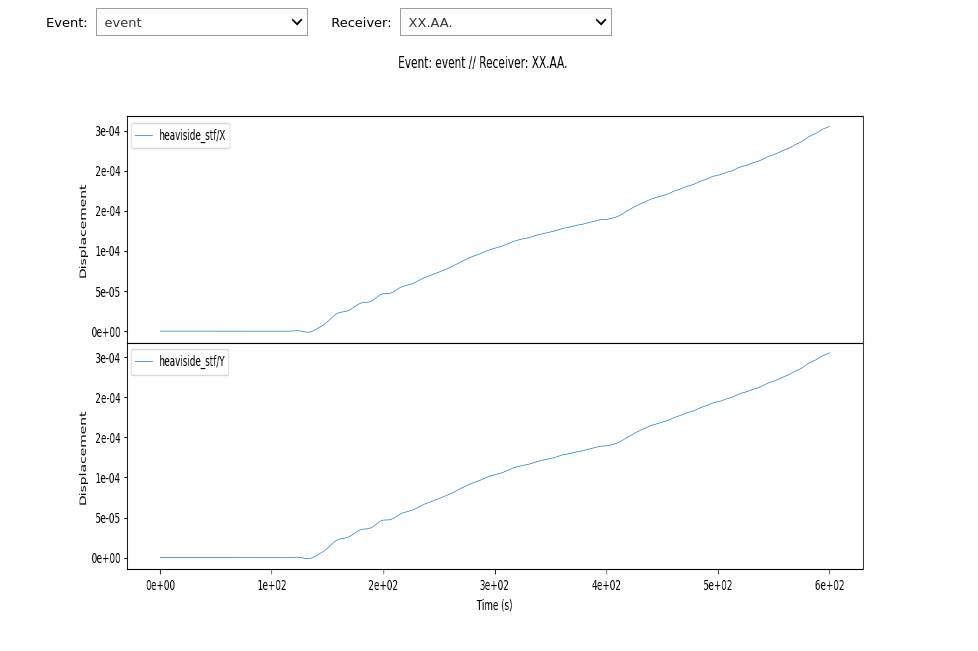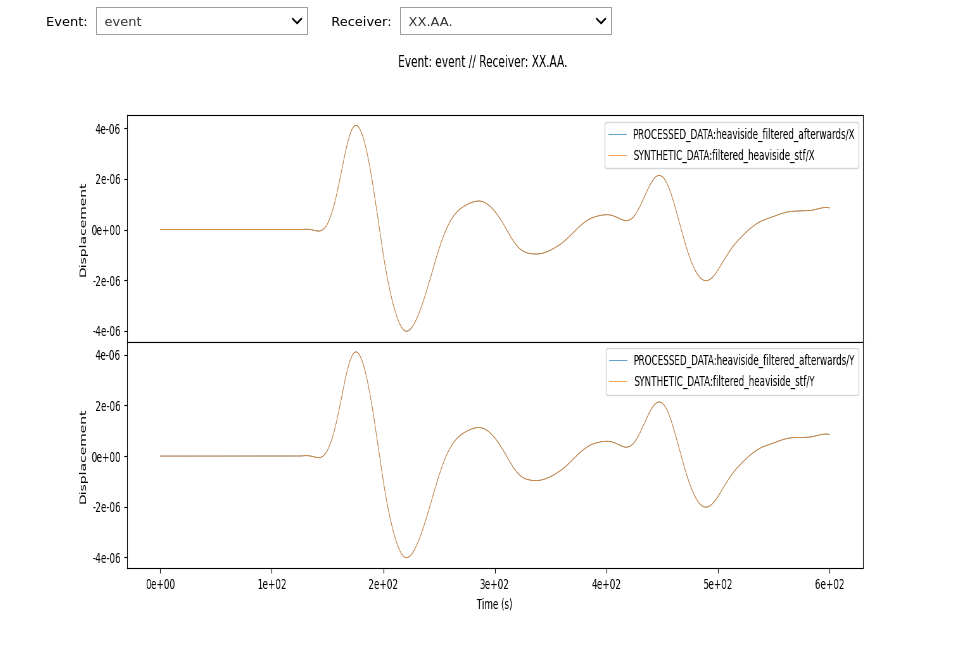Version:

This documentation is not for the latest stable Salvus version.This tutorial is presented as Python code running inside a Jupyter Notebook, the recommended way to use Salvus. To run it yourself you can copy/type each individual cell or directly download the full notebook, including all required files.# Equivalence of Filtering STFs and Filtering Synthetics

This notebook contains a quick demonstration that simulating and then filtering is equivalent to filtering the source time function and then simulating with that.

A case where this matters is when computing adjoint sources during full waveform inversions. Any processing applied to the synthetics will have to be accounted for (because the derivative of the misfit with respect to the original simulation output is required). This can get quite hairy especially when some more advanced processing methods are utilized. A simple way out of this it to just move all the processing to the source time function and filter that. This notebook demonstrates the equivalence of both but the adjoint source is much simpler to calculate when filtering the source time function.

Copy
import pathlib
import os

import salvus.namespace as sn

SALVUS_FLOW_SITE_NAME = os.environ.get("SITE_NAME", "local")
PROJECT_DIR = "project"
# Define common filter parameters so they are consistent
# in both cases.
min_frequency_in_hertz = 1.0 / 120.0
max_frequency_in_hertz = 1.0 / 70.0
highpass_corners = 3
lowpass_corners = 3

For this example we also set the time step for the simulations as well as the filtered Heaviside source time function. This is to make sure both are fully exact but not necessary in practice.

# Project with a large 2-D box domain.
d = sn.domain.dim2.BoxDomain(x0=0, x1=1e6, y0=0, y1=1e6)

if pathlib.Path(PROJECT_DIR).exists():
print("Opening existing project.")
p = sn.Project(path=PROJECT_DIR)
else:
print("Creating new project.")
p = sn.Project.from_domain(path=PROJECT_DIR, domain=d)

# One simulation configuration with a Heaviside STF.
p += sn.SimulationConfiguration(
name="heaviside_stf",
tensor_order=1,
elements_per_wavelength=1.25,
min_period_in_seconds=70.0,
model_configuration=sn.ModelConfiguration(
background_model=sn.model.background.homogeneous.IsotropicElastic(
vp=2000, vs=1000.0, rho=1000
)
),
event_configuration=sn.EventConfiguration(
# Heaviside.
wavelet=sn.simple_config.stf.Heaviside(),
waveform_simulation_configuration=sn.WaveformSimulationConfiguration(
end_time_in_seconds=600.0, time_step_in_seconds=2.0
),
),
)

# Another one with a filtered Heaviside STF. Salvus has a convenience
# function for that.
p += sn.SimulationConfiguration(
name="filtered_heaviside_stf",
tensor_order=1,
elements_per_wavelength=1.25,
min_period_in_seconds=70.0,
model_configuration=sn.ModelConfiguration(
background_model=sn.model.background.homogeneous.IsotropicElastic(
vp=2000, vs=1000.0, rho=1000
)
),
event_configuration=sn.EventConfiguration(
# Filtered Heaviside.
wavelet=sn.simple_config.stf.FilteredHeaviside(
min_frequency_in_hertz=min_frequency_in_hertz,
max_frequency_in_hertz=max_frequency_in_hertz,
end_time_in_seconds=600.0,
highpass_corners=highpass_corners,
lowpass_corners=lowpass_corners,
sampling_rate_in_hertz=0.5,
),
waveform_simulation_configuration=sn.WaveformSimulationConfiguration(
end_time_in_seconds=600.0, time_step_in_seconds=2.0
),
),
)

# Single source + receiver.
p += sn.Event(
event_name="event",
sources=[
sn.simple_config.source.cartesian.VectorPoint2D(
x=0.5e6, y=0.5e6, fx=1e6, fy=1e6
)
],
receivers=[
sn.simple_config.receiver.cartesian.Point2D(
x=0.7e6, y=0.7e6, station_code="AA", fields=["displacement"]
)
],
)

# Launch both
for sim in ["heaviside_stf", "filtered_heaviside_stf"]:
p.simulations.launch(
sim,
p.events.list(),
site_name=SALVUS_FLOW_SITE_NAME,
ranks_per_job=2,
)
p.simulations.query(block=True)
Creating new project.
[2021-03-02 12:44:01,380] INFO: Creating mesh. Hang on.
[2021-03-02 12:44:01,701] INFO: Submitting job ...
Uploading 1 files...

🚀  Submitted [email protected]local
[2021-03-02 12:44:01,923] INFO: Submitting job ...
Uploading 2 files...

🚀  Submitted [email protected]localTrue

The raw simulations with the Heaviside STF will look bad.

p.viz.nb.waveforms(["heaviside_stf"], receiver_field="displacement")We need to filter them to be able to compare to the filtered Heaviside one. We'll utilize a helper function here that calls a Butterworth highpass and a subsequent lowpass filter in exactly the same way as used in the FilteredHeaviside source time function.

from salvus.project.tools.processing import (
get_bandpass_filter_processing_function,
)

p.add_to_project(
sn.processing.ProcessingConfiguration(
name="heaviside_filtered_afterwards",
data_source_name="heaviside_stf",
# Could use a custom function but this yields a fully
# compatible processing function.
processing_function=get_bandpass_filter_processing_function(
min_frequency_in_hertz=min_frequency_in_hertz,
max_frequency_in_hertz=max_frequency_in_hertz,
highpass_corners=highpass_corners,
lowpass_corners=lowpass_corners,
),
),
overwrite=True,
)

# Now this already looks much better.
p.viz.nb.waveforms(
[
"PROCESSED_DATA:heaviside_filtered_afterwards",
"SYNTHETIC_DATA:filtered_heaviside_stf",
],
receiver_field="displacement",
)# Let's do a final numerical equality check.
import numpy as np

st1 = p.waveforms.get(
"SYNTHETIC_DATA:filtered_heaviside_stf", events=p.events.list()
).get_receiver_data("XX.AA.", receiver_field="displacement")
st2 = p.waveforms.get(
"PROCESSED_DATA:heaviside_filtered_afterwards", events=p.events.list()
).get_receiver_data("XX.AA.", receiver_field="displacement")

for tr1, tr2 in zip(st1, st2):
# We have to give some tolerance for numerical inaccuracies.
np.testing.assert_allclose(
tr1.data, tr2.data, rtol=1e-3, atol=1e-5 * np.abs(tr1.data).max()
)PAGE CONTENTS